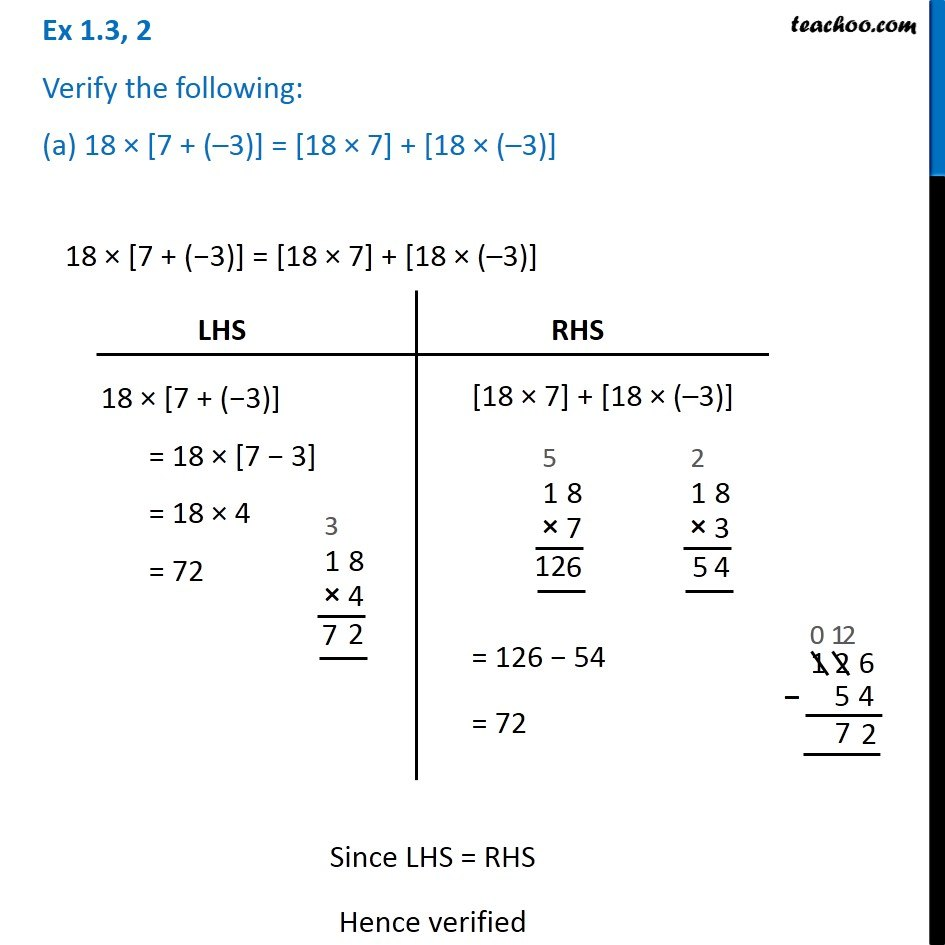1. Chapter 1 Class 7 Integers
2. Concept wise
3. Distributive law in Integers

Transcript

Ex 1.3, 2 Verify the following: (a) 18 × [7 + (–3)] = [18 × 7] + [18 × (–3)] 18 × [7 + (−3)] = [18 × 7] + [18 × (–3)] LHS 18 × [7 + (−3)] = 18 × [7 − 3] = 18 × 4 = 72 RHS [18 × 7] + [18 × (–3)] = 126 − 54 = 72 Since LHS = RHS Hence verified